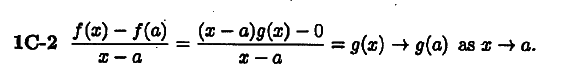# Show that f'(a) = g(a) ?

## Homework Statement

Problem:
1C-2 Let f(x) = (x - a)g(x). Use the definition of the derivative to calculate that f'(a) = g(a), assuming that g is continuous

## The Attempt at a Solution

I just started a self study of calculus using Khan and MIT OpenCourse. I am bit stuck, or maybe confused, with this exercise from MIT 18.01

The definition for the derivative is
$\frac{Δy}{Δx}$=$\frac{f(x)-f(a)}{x-a}$

I can replace f(x) with (x-a)g(x), but I don't know what to do with f(a). In the given solution f(a) somehow becomes 0, but I can't see how.

The given solution:Any help is much appreciated.

## Answers and Replies

LCKurtz
Science Advisor
Homework Helper
Gold Member
f(a) = (a-a)g(a) = 0.

ah - of course. Thank you very much